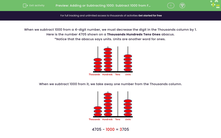# Subtract 1,000 from a Four-Digit Number

In this worksheet, students will subtract 1,000 from a four-digit number.Key stage:  KS 2

Curriculum topic:   Number: Number and Place Value

Curriculum subtopic:   Find 1000 More or Less than a Given Number

Difficulty level:#### Worksheet Overview

When we subtract 1,000 from a four-digit number, we need to decrease the digit in the thousands column by 1.

Here is the number 4,705 shown on an abacus.

Notice that the abacus says units. Units are another word for ones.When we subtract 1,000 from it, we take away one digit from the thousands column.4,705 - 1,000 = 3,705

Example 1

Subtract 1,000 from:

5,097

We decrease the 5 in the thousands column by 1 to get 4 in that column.

5,097 - 1,000 = 4,097

Example 2

Subtract 1,000 from:

1,060

We decrease the 1 in the thousands column by 1 to get 0 in that column.

Remember to leave off any zeros on the left-hand side of a number.

1,060 - 1,000 = 0060 = 60

Does that make sense?Let's get started!

### What is EdPlace?

We're your National Curriculum aligned online education content provider helping each child succeed in English, maths and science from year 1 to GCSE. With an EdPlace account you’ll be able to track and measure progress, helping each child achieve their best. We build confidence and attainment by personalising each child’s learning at a level that suits them.

Get started